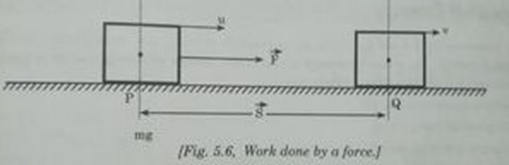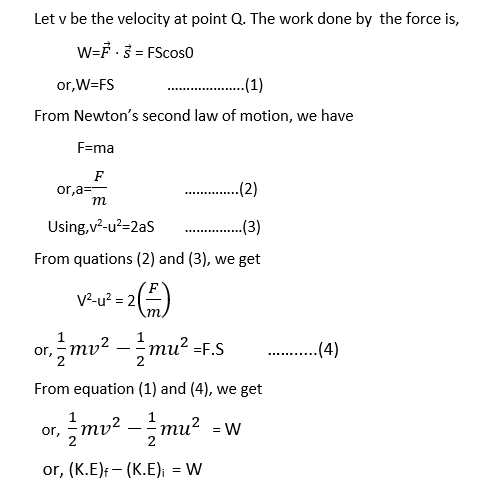# Work-Energy TheoremWork-Energy theorem states that “the work done on a body by a resultant force is equal to the change in kinetic energy of the body.”

Suppose a body having mass ‘m’ is moving initially with velocity ‘u’ at point P. Let a constant force ‘F’ is applied to the body and the body covers a displacement ‘S'

Form point P to Q  as shown in the figure:This equation shows that the workforce on moving a body is equal to change in kinetic energy of the body. This proves the work-energy theorem.